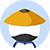If $wxy \neq 0$, does x = y ?

(1) $w^x = w^y$

(2) $wxy \neq xy$
• AStatement 1 ALONE is sufficient to answer the question, but statement 2 alone is NOT sufficient.
• BStatement 2 ALONE is sufficient to answer the question, but statement 1 alone is NOT sufficient.
• CBOTH statements 1 and 2 TOGETHER are sufficient to answer the question, but NEITHER statement ALONE is sufficient.
• DEach statement ALONE is sufficient to answer the question.
• EStatement 1 and 2 TOGETHER are NOT sufficient to answer the question. Previous

• 网友解析

### 题目讨论

• 优质讨论
• 最新讨论• 所属科目:数学DS
• 题目来源1:Magoosh-227
• 正确率: 32%
• 难度: